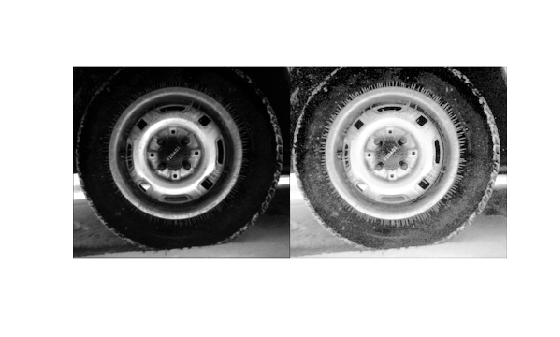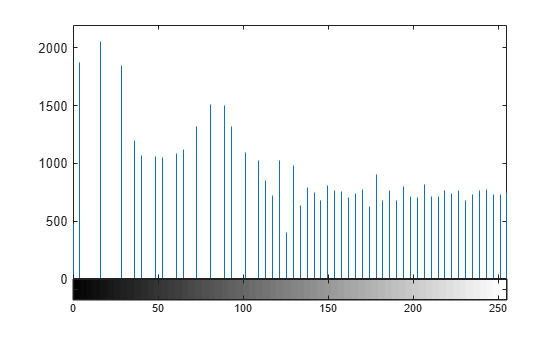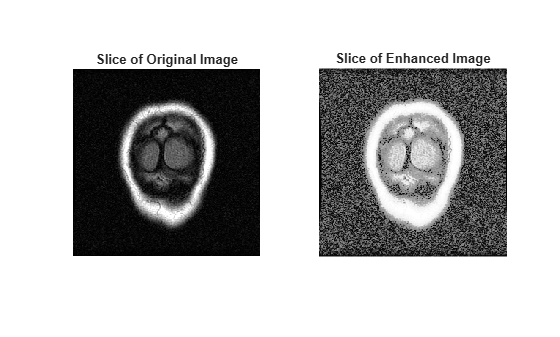# histeq

Enhance contrast using histogram equalization

## Syntax

``````J = histeq(I)``````
``J = histeq(I,n)``
``J = histeq(I,hgram)``
``newcmap = histeq(X,map)``
``newcmap = histeq(X,map,hgram)``
``````[___,T] = histeq(___)``````

## Description

example

``````J = histeq(I)``` transforms the grayscale image `I` so that the histogram of the output grayscale image `J` has 64 bins and is approximately flat.```
````J = histeq(I,n)` transforms the grayscale image `I` so that the histogram of the output grayscale image `J` with `n` bins is approximately flat. The histogram of `J` is flatter when `n` is much smaller than the number of discrete levels in `I`. ```
````J = histeq(I,hgram)` transforms the grayscale image `I` so that the histogram of the output grayscale image `J` approximately matches the target histogram `hgram`. The number of bins in the histogram of the output image is equal to `length(hgram)`.```
````newcmap = histeq(X,map)` transforms the values in the colormap so that the histogram of the gray component of the indexed image `X` is approximately flat. The transformed colormap is `newcmap`.```
````newcmap = histeq(X,map,hgram)` transforms the colormap associated with the indexed image `X` so that the histogram of the gray component of the indexed image (`X`,`newcmap`) approximately matches the target histogram `hgram`. The `histeq` function returns the transformed colormap in `newcmap`. `length(hgram)` must be the same as `size(map,1)`.```

example

``````[___,T] = histeq(___)``` also returns the transformation `T` that maps the gray component of the input grayscale image or colormap to the gray component of the output grayscale image or colormap.```

## Examples

collapse all

Read an image into the workspace.

`I = imread('tire.tif');`

Enhance the contrast of an intensity image using histogram equalization.

`J = histeq(I);`

Display the original image and the adjusted image.

```imshowpair(I,J,'montage') axis off```Display a histogram of the original image.

```figure imhist(I,64)```Display a histogram of the processed image.

```figure imhist(J,64)````load mristack`

Perform histogram equalization.

`enhanced = histeq(mristack);`

Display the first slice of data for the original image and the contrast-enhanced image.

```figure subplot(1,2,1) imshow(mristack(:,:,1)) title('Slice of Original Image') subplot(1,2,2) imshow(enhanced(:,:,1)) title('Slice of Enhanced Image')```This example shows how to plot the transformation curve for histogram equalization. `histeq` can return a 1-by-256 vector that shows, for each possible input value, the resulting output value. (The values in this vector are in the range [0,1], regardless of the class of the input image.) You can plot this data to get the transformation curve.

`I = imread('pout.tif');`

Adjust the contrast using histogram equalization, using the `histeq` function. Specify the gray scale transformation return value, `T`, which is a vector that maps graylevels in the intensity image `I` to gray levels in `J`.

`[J,T] = histeq(I);`

Plot the transformation curve. Notice how this curve reflects the histograms in the previous figure, with the input values mostly between 0.3 and 0.6, while the output values are distributed evenly between 0 and 1.

```figure plot((0:255)/255,T);```## Input Arguments

collapse all

Grayscale image, specified as a numeric array of any dimension.

Data Types: `single` | `double` | `int16` | `uint8` | `uint16`

Target histogram, specified as a numeric vector. The target histogram must have equally spaced bins spanning intensity values in the appropriate range. The intensity value range depends on the data type of the input image.

• `single` or `double` — [0, 1].

• `uint8` — [0, 255].

• `uint16` — [0, 65535].

• `int16` — [–32768, 32767].

By default, the number of bins in the target histogram is equal to `length(hgram)`.

For example:

```hgram = 256:-4:4; J = histeq(I,hgram);```
specifies a target histogram with 64 bins as a non-flat, linearly decreasing function which emphasizes small pixel values.

`histeq` automatically scales `hgram` so that `sum(hgram) == numel(I)`. The histogram `J` better matches `hgram` when `length(hgram)` is much smaller than the number of discrete levels in `I`.

Data Types: `single` | `double`

Number of discrete gray levels, specified as a positive integer.

Data Types: `single` | `double`

Indexed image, specified as a numeric array of any dimension. The values in `X` are an index into the colormap `map`.

Data Types: `single` | `double` | `uint8` | `uint16`

Colormap associated with indexed image `X`, specified as a c-by-3 numeric matrix with values in the range [0, 1]. Each row is a three-element RGB triplet that specifies the red, green, and blue components of a single color of the colormap.

Data Types: `double`

## Output Arguments

collapse all

Transformed grayscale image, returned as a numeric array of the same size and class as the input image `I`.

Grayscale transformation, returned as a numeric vector. The transformation `T` maps gray levels in the image `I` to gray levels in `J`.

Data Types: `double`

Transformed colormap, specified as an n-by-3 numeric matrix with values in the range [0, 1]. Each row is a three-element RGB triplet that specifies the red, green, and blue components of a single color of the colormap.

Data Types: `double`

## Algorithms

When you supply a target histogram `hgram`, `histeq` chooses the grayscale transformation `T` to minimize

`$|{c}_{1}\left(T\left(k\right)\right)-{c}_{0}\left(k\right)|,$`

c0 is the cumulative histogram of the input image `I`, and c1 is the cumulative sum of `hgram` for all intensities k. This minimization is subject to these constraints:

• `T` must be monotonic

• c1(T(a)) cannot overshoot c0(a) by more than half the distance between the histogram counts at a

`histeq` uses the transformation b = T(a) to map the gray levels in `X` (or the colormap) to their new values.

If you do not specify `hgram`, then `histeq` creates a flat `hgram`,

`hgram = ones(1,n)*prod(size(A))/n;`

and then applies the previous algorithm.

## Version History

Introduced before R2006a

expand all